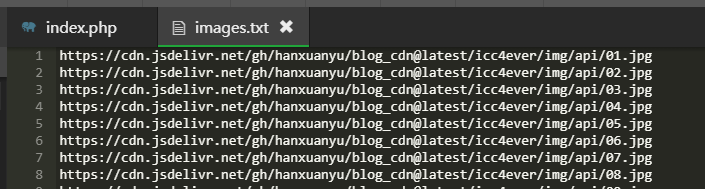# 制作方法

• 首先就是准备你喜欢的高清图片了，可以在网络上寻找喜欢的图片，然后将这些图片放在服务器上的某文件夹内，当然也可以部署在cdn上以加快访问速度。
• 图片准备好后，网站目录新建一个文件夹，并在文件夹下新建index.php文件。index.php中的内容如下：
<?php
//存有图片链接的文件名images.txt
$filename = "images.txt"; if(!file_exists($filename)){
die('指定文件不存在(images.txt)');
}

//从文本获取链接
$pics = [];$fs = fopen($filename, "r"); while(!feof($fs)){
$line=trim(fgets($fs));
if($line!=''){ array_push($pics, $line); } } //从数组随机获取链接$pic = $pics[array_rand($pics)];

//返回指定格式
$type=$_GET['type'];
switch($type){ //JSON返回 case 'json': header('Content-type:text/json'); die(json_encode(['pic'=>$pic]));

default:
?>
• 在文件夹下新建一个images.txt文本文档，并在其内部填上图片的url，要求一行一个，格式如下：